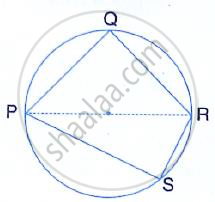Share

# The Following Figure Shows a Circle with Pr as Its Diameter. If Pq = 7 Cm and Qr = 3rs = 6 Cm, Find the Perimeter of the Cyclic Quadrilateral Pqrs. - Mathematics

Course

#### Question

The following figure shows a circle with PR as its diameter.
If PQ = 7 cm and QR = 3RS = 6 cm, find the perimeter of the cyclic quadrilateral PQRS.#### Solution

In the figure, PQRS is a cyclic quadrilateral in which PR is a diameter
PQ = 7 cm
QR = 3 RS = 6 cm
3 RS = 6 cm ⇒ RS = 2 cm

Now in ∆PQR,
∠Q = 90°        [Angles in a semi circle]

PR^ 2 = PQ^2 + QR^2 [Pythagoras theorem]

= 72^2 + 62^2
= 49 + 36
= 85

Again in right= ΔPSQ,  PR^2 =PS^2 + RS^2

85 = PS^2 + 2^2

PS^2 = 85 - 4 = 81 = (9)^2
PS = 9cm
Now, perimeter of quad PQRS = PQ + QR + RS + SP
= (7 + 9 + 6 )cm
= 24

Is there an error in this question or solution?